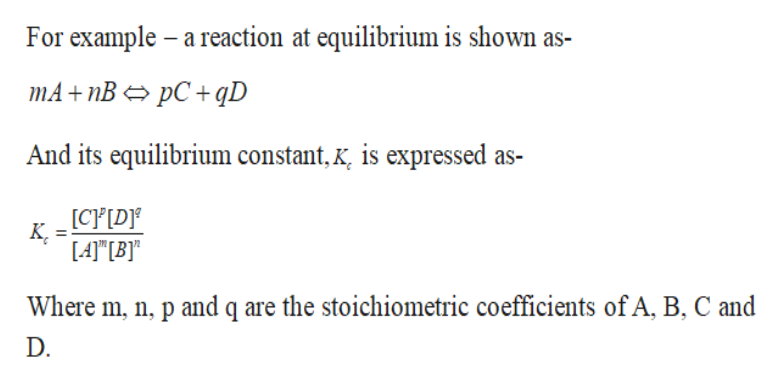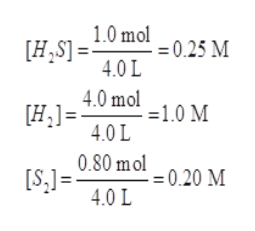On analysis, an equilibrium mixture for the reaction 2H2S(g) 2H2(g) + S2(g)was found to contain 1.0 mol H2S, 4.0 mol H2, and 0.80 mol S2 in a 4.0L vessel.Calculate the equilibrium constant, Kc, for this reaction.

Question

On analysis, an equilibrium mixture for the reaction
2H2S(g) 2H2(g) + S2(g)
was found to contain 1.0 mol H2S, 4.0 mol H2, and 0.80 mol S2 in a 4.0L vessel.
Calculate the equilibrium constant, Kc, for this reaction.

Step 1

Equilibrium constant-

It is defined as the ratio of the equilibrium concentration of the products raised to the power of their stoichiometric coefficients to the equilibrium concentration of the reactants raised to the power of their stoichiometric coefficients.help_outlineImage TranscriptioncloseFor example a reaction at equilibrium is shown as- mA+ пB > pC + qD And its equilibrium constant, k, is expressed as- [CP[D К, LA B) Where m, n, p and q are the stoichiometric coefficients of A, B, C and D. fullscreen
Step 2

The given reaction mixture has 1.0 mol H2S, 4.0 mol H2, and 0.80 mol S2 in a 4.0L vessel. We have to determine the equilibrium constant for the given reaction.

Step 3

Molarity is defined as the moles of solute dissolved per litre of solution.

Given- At equilibrium:

Moles of H2S = 1.0

Moles ...help_outlineImage Transcriptionclose1.0 mol025 M [H,S]- 4.0 L [H=40 mol 1.0 M 4.0 L 0.80 mol0.20 M [S,l=40L fullscreen

Want to see the full answer?

See Solution

Want to see this answer and more?

Our solutions are written by experts, many with advanced degrees, and available 24/7

See Solution
Tagged in

General Chemistry